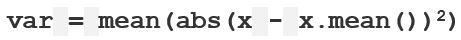# numpy.nanvar() in Python

`numpy.nanvar(arr, axis = None)` : Compute the variance of the given data (array elements) along the specified axis(if any), while ignoring NaN values.Example :

x = 1 1 1 1 1
Standard Deviation = 0 . Variance = 0

y = 9, 2, 5, 4, 12, 7, 8, 11, 9, 3, 7, 4, 12, 5, 4, 10, 9, 6, 9, 4

Step 1 : Mean of distribution 4 = 7
Step 2 : Summation of (x – x.mean())**2 = 178
Step 3 : Finding Mean = 178 /20 = 8.9
This Result is Variance.

Parameters :
arr : [array_like] input array.
axis : [int or tuples of int]axis along which we want to calculate the variance. Otherwise, it will consider `arr `to be flattened (works on all the axis). axis = 0 means variance along the column and axis = 1 means variance along the row.
out : [ndarray, optional]Different array in which we want to place the result. The array must have the same dimensions as expected output.
dtype : [data-type, optional]Type we desire while computing variance.

Results : Variance of the array (a scalar value if axis is none) or array with variance values along specified axis; while ignoring NaN values.

Code #1:

 `# Python Program illustrating  ` `# numpy.nanvar() method  ` `import` `numpy as np  ` `     `  `# 1D array  ` `arr ``=` `[``20``, ``2``, np.nan, ``1``, ``34``]  ` ` `  `print``(``"arr : "``, arr)  ` `print``(``"\nnanvar of arr : "``, np.nanvar(arr))  ` ` `  `print``(``"var of arr : "``, np.var(arr))  ` ` `  `print``(``"\nnanvar of arr : "``, np.nanvar(arr, dtype ``=` `np.float32))  ` `print``(``"var of arr : "``, np.var(arr, dtype ``=` `np.float32))  ` ` `

Output :

```arr :  [20, 2, nan, 1, 34]

nanvar of arr :  187.1875
var of arr :  nan

nanvar of arr :  187.1875
var of arr :  nan```

Code #2:

 `# Python Program illustrating  ` `# numpy.nanvar() method  ` `import` `numpy as np  ` `     `  ` `  `# 2D array  ` `arr ``=` `[[``2``, ``2``, ``2``, ``2``, ``2``],  ` `    ``[``15``, ``6``, np.nan, ``8``, ``2``],  ` `    ``[``23``, ``2``, ``54``, ``1``, ``2``, ],  ` `    ``[np.nan, ``44``, ``34``, ``7``, ``2``]]  ` ` `  `     `  `# nanvar of the flattened array  ` `print``(``"\nnanvar of arr, axis = None : "``, np.nanvar(arr))  ` ` `  `print``(``"\nvar of arr, axis = None : "``, np.var(arr))  ` ` `  `     `  `# nanvar along the axis = 0  ` `print``(``"\nnanvar of arr, axis = 0 : \n"``, np.nanvar(arr, axis ``=` `0``))  ` ` `  `print``(``"\nvar of arr, axis = 0 : "``, np.var(arr, axis ``=` `0``))  ` ` `  `# nanvar along the axis = 1  ` `print``(``"\nnanvar of arr, axis = 1 : "``, np.nanvar(arr, axis ``=` `1``))  `

Output :

```nanvar of arr, axis = None :  249.88888888888889

var of arr, axis = None :  nan

nanvar of arr, axis = 0 :
[ 74.88888889 312.75       458.66666667   9.25         0.        ]

var of arr, axis = 0 :  [   nan 312.75    nan   9.25   0.  ]

nanvar of arr, axis = 1 :  [  0.      22.1875 421.84   313.1875]```

My Personal Notes arrow_drop_upIf you like GeeksforGeeks and would like to contribute, you can also write an article using contribute.geeksforgeeks.org or mail your article to contribute@geeksforgeeks.org. See your article appearing on the GeeksforGeeks main page and help other Geeks.

Please Improve this article if you find anything incorrect by clicking on the "Improve Article" button below.

Article Tags :

Be the First to upvote.

Please write to us at contribute@geeksforgeeks.org to report any issue with the above content.